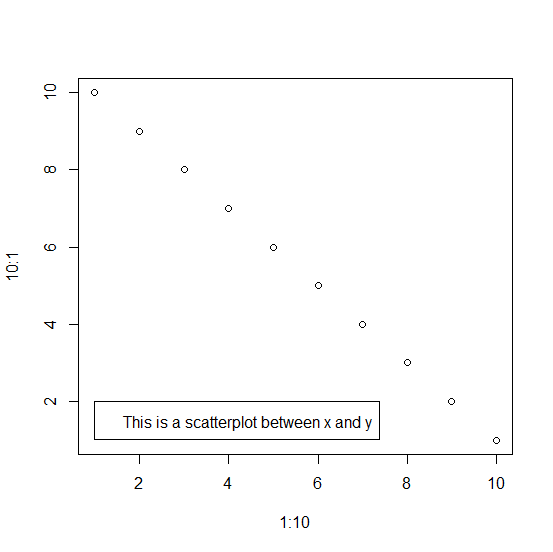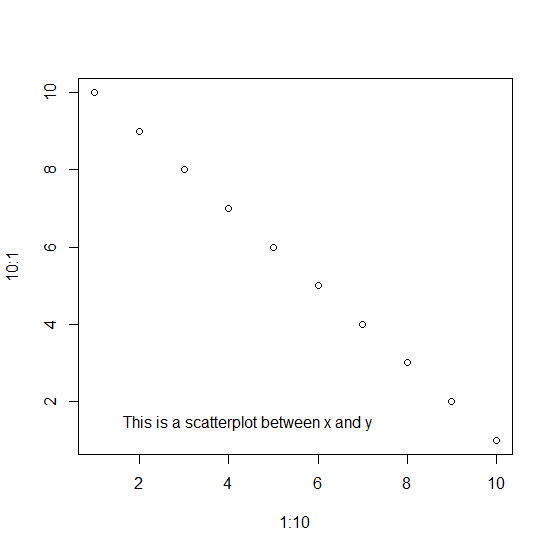# How to remove the border of the legend of a chart created by plot function in R?

When we create a chart using plot function and add a legend to that chart, the output of the chart has the legend that is covered with borders. But this breaks the flow of the chart and the area covered by the order make the chart unattractive. Therefore, we can use bty="n" with the legend function and it will remove the border of the legend.

## Example

Creating the chart with legend that has border −

plot(x=1:10,y=10:1)
legend(1,2,"This is a scatterplot between x and y")

## OutputCreating the chart with legend which does not have a border −

## Example

plot(x=1:10,y=10:1)
legend(1,2,"This is a scatterplot between x and y",bty="n")

## Output